Courses

# Test: Give And Take - 2

## 20 Questions MCQ Test Mathematics for Class 2: NCERT | Test: Give And Take - 2

Description
This mock test of Test: Give And Take - 2 for Class 2 helps you for every Class 2 entrance exam. This contains 20 Multiple Choice Questions for Class 2 Test: Give And Take - 2 (mcq) to study with solutions a complete question bank. The solved questions answers in this Test: Give And Take - 2 quiz give you a good mix of easy questions and tough questions. Class 2 students definitely take this Test: Give And Take - 2 exercise for a better result in the exam. You can find other Test: Give And Take - 2 extra questions, long questions & short questions for Class 2 on EduRev as well by searching above.
QUESTION: 1

Solution:
QUESTION: 2

### How many tens are there in the sum of 570 and 430?

Solution: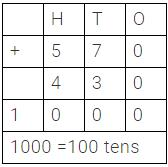QUESTION: 3

### What comes next in the given series?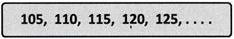Solution:

125 + 5 = 130

QUESTION: 4

Which digit in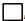, makes the addition correct?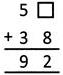Solution:
QUESTION: 5

Study the pattern and fill the missing term.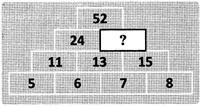Solution:

13 + 15 = 28

QUESTION: 6

There are 3 similar dice as shown. The numbers on the faces of each of the dice are 10, 20, 30, 40, 50 or 60. Jack throws all the three dice at once. The total is 130.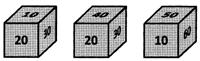What is the number on each of the dice if the difference between the smallest and the biggest numbers is 40?

Solution:
QUESTION: 7

How many corners does this shape have?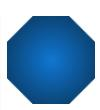Solution:

8 corners

QUESTION: 8

Which number should be written in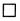to make the given number sentence correct?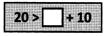Solution:

5 + 10 = 15
= 20 > 15
So, 5 must be written in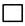.

QUESTION: 9

The first four figures in a pattern are as shown.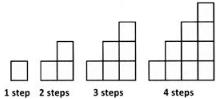If the pattern continues in this way, how many blocks are needed to make 6 steps?

Solution:

1 + 2 + 3 + 4 + 5 + 6 = 21

QUESTION: 10

If we add 9 tens, 5 hundreds and 3 ones, then what is the result?

Solution:

5 hundreds + 9 tens + 3 ones
= 500 + 90 + 3 = 593

QUESTION: 11

A number machine takes any number put into it, adds 20, then subtracts 10, and then adds 8.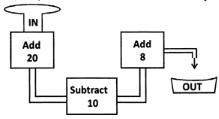If 13 is put into the machine, what number comes out of it?

Solution:

13 + 20 = 33
33 - 10 = 23
23 + 8 = 31

QUESTION: 12

Which number should fill the box to make the number sentence correct?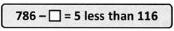Solution:

5 less than 116 is 116 - 5 = 111
786 -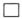=111 means 786 - 111 == 675

QUESTION: 13

What is the difference of 6 tens and 4?

Solution:

6 tens = 60  60 - 4 = 56

QUESTION: 14

The two number sentences shown are true.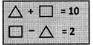Which of the following are the possible values of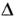and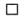?

Solution: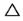+= 10-= 2 are true.
Taking the values Ofandfrom the given options,= 6 and= 4 as
6 + 4 = 10 and
6 - 4 = 2 are true.

QUESTION: 15

What is the value of N? N - 442 = 382

Solution:

To find the required number add 382 and 442. 382 + 442 = 824 So, 382 is 442 less than 824.

QUESTION: 16

If the smallest one digit counting number is added to a number, which number is got as the sum?

Solution:

The smallest one digit counting number is 1. When 1 is added to a number, the sum is its successor.

QUESTION: 17

Add me to 6 or subtract me from 14. The answer is the same. Who am I?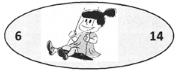Solution:

6 + 4 = 10 = 14 - 4

QUESTION: 18

Which statement is correct?

Solution:

e.g., 732 > 96

QUESTION: 19

Look at the picture given.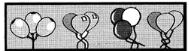Which of the following number sentences can describe the given picture?

Solution:

Each bunch has 3 balloons.
So, the total number of balloons = 3 + 3 + 3 + 3 = 12.

QUESTION: 20

By how much is 688 more than 480?

Solution:

688 - 480 = 208
So, 688 is 208 more than 480.

Track your progress, build streaks, highlight & save important lessons and more!

### Similar Content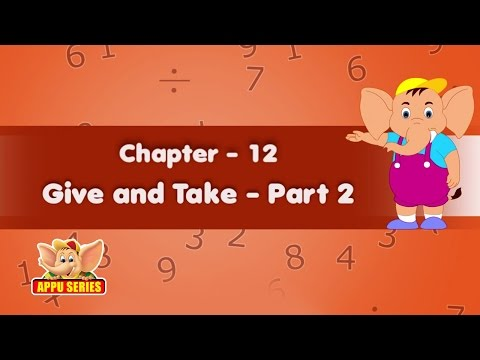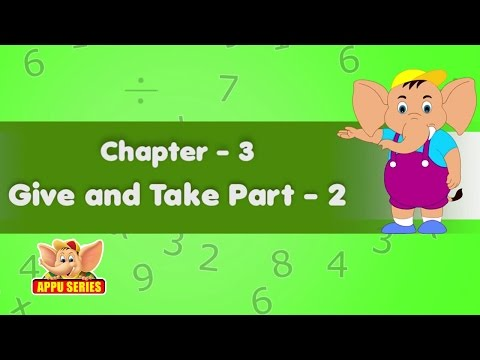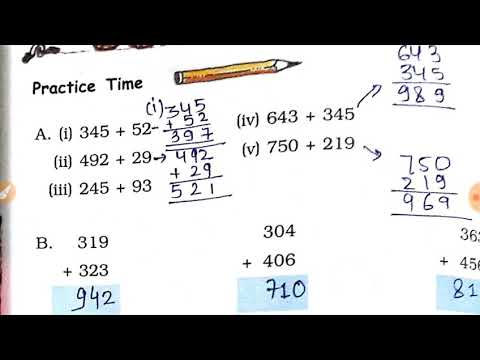### Related tests